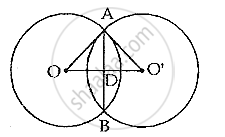Share

# The Length of Common Chord of Two Intersecting Circles is 30 Cm. If the Diameters of These Two Circles Be 50 Cm and 34 Cm, Calculate the Distance Between Their Centres. - Mathematics

Course

#### Question

The length of common chord of two intersecting circles is 30 cm. If the diameters of these two circles be 50 cm and 34 cm, calculate the distance between their centres.

#### SolutionOA = 25 cm and AB = 30 cm

∴ AD = 1/2× AB =(1/2×30)cm = 15 cm

OA^2 = AD^2 + OD^2
⇒ OD^2 = OA^2 - OD^2 = 25^2 -15^2
= 625 - 225 = 400

∴ OD =sqrt 400 = 20 cm
Again, we have O 'A = 17 cm
 O'A ^2= AD^2 +O'D^2
⇒ O'D^2 =O'A^2 - AD^2 = 17^2 -15^2
⇒ 289 - 225 = 64
∴ O'D 8 cm
∴ OO' (OD+ O'D)
= (20 +8)= 28 cm
∴ the distance between their centres is 28 cm

Is there an error in this question or solution?